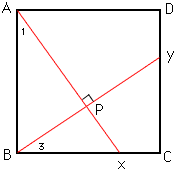My name is Monica I am a 12th grade student. My problem is in geometry. Given: ABCD is a square; AX is perpendicular to BY Prove: Angle 1 is congruent to Angle 3 Hi Monica, Label the point of intersection of AX and BY as P, as in the diagram below.Since triangle ABP is a right angle triangle, and the three angles in any triangle sum to 180o, angle ABP + angle 1 = 90o. Since angle ABX is a right angle, angle ABP + angle 3 = 90o. Thus angle 1 = angle 3. Cheers, Chris Go to Math Central